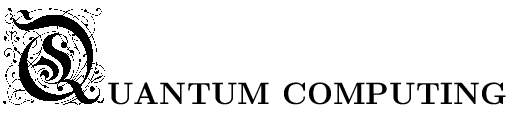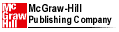Jozef Gruska

Leaflet

ISBN: 0-07-709503-0
Published: May 1999
Binding:  Softcover
Pages: 439 - 246x189
Price: Ł34.99, DM73

## Contents

Contents
Preface
1. FUNDAMENTALS
1. Why Quantum Computing
2. Prehistory of Quantum Computing
3. From Randomized to Quantum Computation
1. Probabilistic Turing machines
2. Quantum Turing machines
4. Hilbert Space Basics
1. Orthogonality, bases and subspaces
2. Operators
3. Observables and measurements
4. Tensor products in Hilbert spaces
5. Mixed states and density operators
5. Experiments
1. Classical experiments
2. Quantum experiments---single particle interference
3. Quantum experiments---measurements
6. Quantum Principles
1. States and amplitudes
2. Measurements---the projection approach
3. Evolution of quantum systems
4. Compound quantum systems
5. Quantum theory interpretations
7. Classical Reversible Gates and Computing
1. Reversible gates
2. Reversible Turing machines
3. Billiard ball model of (reversible) computing
2. ELEMENTS
1. Quantum Bits and Registers
1. Qubits
2. Two-qubit registers
3. No-cloning theorem
4. Quantum registers
2. Quantum Entanglement
1. Entanglement of pure states
2. Quantifying entanglement
3. Substituting entanglement for communication
3. Quantum Circuits
1. Quantum gates
2. Measurement gates
3. Universality of quantum gates
4. Arithmetical circuits
5. Quantum superoperator circuits
3. ALGORITHMS
1. Quantum Parallelism and Simple Algorithms
1. Deutsch's problem
2. The Deutsch--Jozsa promise problem
3. Simon's problems
2. Shor's Algorithms
1. Number theory basics
2. Quantum Fourier Transform
3. Shor's factorization algorithm
4. Shor's discrete logarithm algorithm
5. The hidden subgroup problems
3. Quantum Searching and Counting
1. Grover's search algorithm
2. Reflections of Grover's iterate and search principles
3. G-BBHT search algorithm
4. Minimum-finding algorithm
5. Generalizations and modifications of search problems
4. Methodologies to Design Quantum Algorithms
1. Amplitude amplification--boosting search probabilities
2. Amplitude amplification---speeding of the states searching
3. Case studies
5. Limitations of Quantum Algorithms
1. No quantum speed-up for the parity function
2. Framework for proving lower bounds
3. Oracle calls limitation of quantum computing
4. AUTOMATA
1. Quantum Finite Automata
1. Models of classical finite automata
2. One-way quantum finite automata
3. One-measurement model of one-way QFA
4. Equivalence of MO-1QFA and their simulations by PFA
5. 1QFA versus 1FA
6. Two-way quantum finite automata
7. 2QFA versus 1FA
2. Quantum Turing Machines
1. One-tape quantum Turing machines
2. Variations on the basic model
3. Are quantum Turing machines analogue or discrete?
4. Programming techniques for quantum Turing machines
3. Quantum Cellular Automata
1. Classical cellular automata
2. One-dimensional quantum cellular automata
3. Partitioned quantum one-dimensional cellular automata
4. Quantum cellular automata versus quantum Turing machines
5. COMPLEXITY
1. Universal Quantum Turing Machines
1. Efficient implementation of unitary transformations
2. Design of a universal quantum Turing machine
2. Quantum Computational Complexity
1. Basic quantum versus classical complexity classes
2. Relativized quantum complexity
3. Quantum Communication Complexity
1. Classical and quantum communication protocols and complexity
2. Quantum communication versus computation complexity
4. Computational Power of Quantum Non-linear Mechanics
6. CRYPTOGRAPHY
1. Prologue
2. Quantum Key Generation
1. Basic ideas of two parties quantum key generation
2. Security issues of QKG protocols
3. Quantum key generation protocols BB84 and B92
4. Multiparty key generation
5. Entanglement-based QKG protocols
6. Unconditional security of QKG*
7. Experimental quantum cryptography
3. Quantum Cryptographic Protocols
1. Quantum coin-flipping and bit commitment protocols
2. Quantum oblivious transfer protocols
3. Security of the quantum protocols
4. Security limitations of the quantum cryptographic protocols
5. Insecurity of quantum one-sided two-party computation protocols
4. Quantum Teleportation and Superdense Coding
1. Basic principles
2. Teleportation circuit
3. Quantum secret sharing
4. Superdense coding
7. PROCESSORS
1. Early Quantum Computers Ideas
1. Benioff's quantum computer
2. Feynman's quantum computer
3. Peres' quantum computer
4. Deutsch's quantum computer
2. Impacts of Imperfections
1. Internal imperfections
2. Decoherence
3. Quantum Computation and Memory Stabilization
1. The symmetric space
2. Stabilization by projection into the symmetric subspace
4. Quantum Error-Correcting Codes
1. Classical error-detecting and -correcting codes
2. Framework for quantum error-correcting codes
3. Case studies
4. Basic methods to design quantum error-correcting codes
5. Stabilizer codes
5. Fault-tolerant Quantum Computation
1. Fault-tolerant quantum error correction
2. Fault-tolerant quantum gates
3. Concatenated coding
6. Experimental Quantum Processors -
1. Main approaches
2. Ion trap
3. Cavity QED
4. Nuclear magnetic resonance (NMR)
5. Other potential technologies
8. INFORMATION
1. Quantum Entropy and Information
1. Basic concepts of classical information theory
2. Quantum entropy and information
2. Quantum Channels and Data Compression
1. Quantum sources, channels and transmissions
2. Shannon's coding theorems
3. Schumacher's noiseless coding theorem
4. Dense quantum coding
5. Quantum Noisy Channel Transmissions
6. Capacities of erasure and depolarizing channels
3. Quantum Entanglement
1. Transformation and the partial order of entangled states.
2. Entanglement purification/distillation
3. Entanglement concentration and dilution
4. Quantifying entanglement
5. Bound entanglement
4. Quantum Information Processing Principles and Primitives
1. Search for quantum information principles
2. Quantum information processing primitives
9. APPENDIX
1. Quantum Theory
1. Pre-history of quantum theory
2. Heisenberg's uncertainty principle
3. Quantum theory versus physical reality
4. Quantum measurements
7. Interpretations of quantum theory
8. Incompleteness of quantum mechanics
2. Hilbert Space Framework for Quantum Computing
1. Hilbert spaces
2. Linear operators
3. Mixed states and density matrices
4. Probabilities and observables
5. Evolution of quantum states
6. Measurements
7. Tensor products and Hilbert spaces
8. Generalized measurements-POV measurements
3. Deterministic and Randomized Computing
1. Computing models
2. Randomized computations
3. Complexity classes
4. Computational theses
4. Exercises
5. Historical and Bibliographical References
Bibliography
Index